# Dancing zeros

Playing with Matlab, I plotted the location of the zeros of a polynomial with normally distributed coefficients in the complex plane. It was nearly a circle:

This did not surprise me that much, since I have already toyed with the distribution of zeros of polynomials with coefficients in {-1,0,+1}, producing some neat distributions close to the unit circle (see also John Baez). A quick google found (Hughes & Nikeghbali 2008): under very general circumstances polynomial zeros tend towards the unit circle. One can heuristically motivate it in a lot of ways.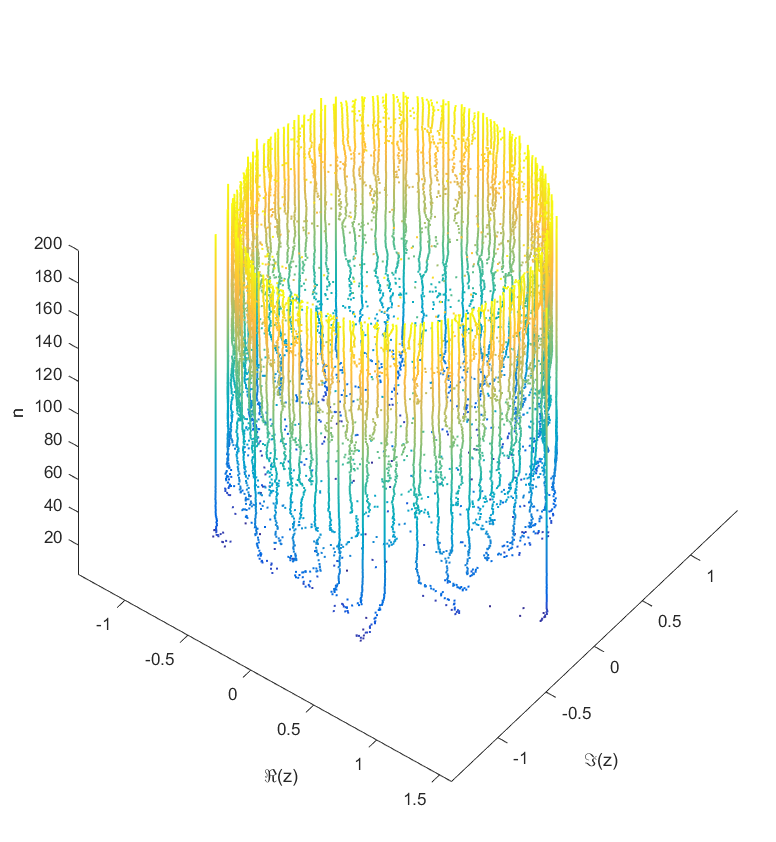Locations of the zeros of a polynomial with a given sequence of normally distributed coefficients, as a function of degree.

As you add more and more terms to the polynomial the zeros approach the unit circle. Each new term perturbs them a bit: at first they move around a lot as the degree goes up, but they soon stabilize into robust positions (“young” zeros move more than “old” zeros). This seems to be true regardless of whether the coefficients set in “little-endian” or “big-endian” fashion.

But then I decided to move things around: what if the coefficient on the leading term changed? How would the zeros move? I looked at the polynomial$P_\theta(z)=e^{i\theta} z^n + c_{n-1}z^{n-1}+\ldots+c_1 z + c_0$ where$c_i$ were from some suitable random sequence and$\theta$ could run around$[0,2\pi]$. Since the leading coefficient would start and end up back at 1, I knew all zeros would return to their starting position. But in between, would they jump around discontinuously or follow orderly paths?

Continuity is actually guaranteed, as shown by (Harris & Martin 1987). As you change the coefficients continuously, the zeros vary continuously too. In fact, for polynomials without multiple zeros, the zeros vary analytically with the coefficients.

As$\theta$ runs from 0 to$2\pi$ the roots move along different orbits. Some end up permuted with each other.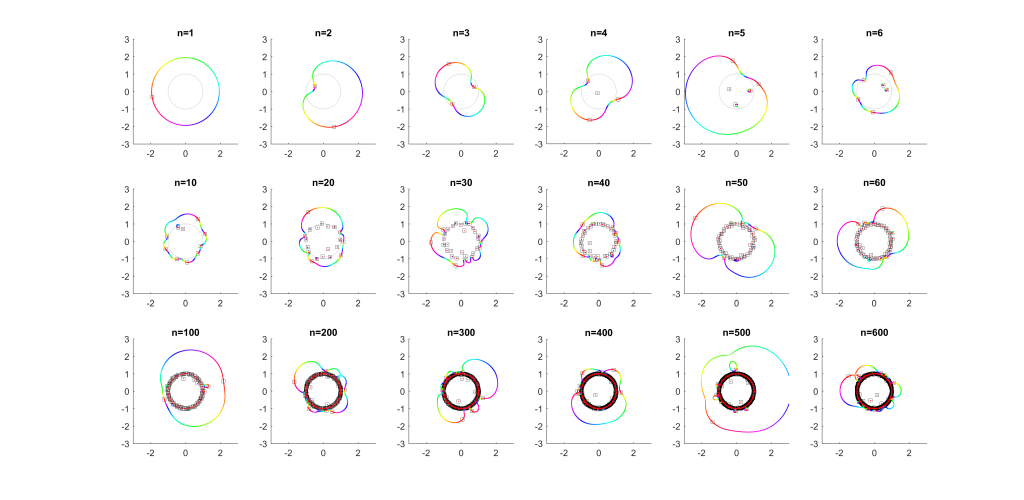Movement of the zeros of polynomials with random coefficients as the leading coefficient traverses the unit circle. Colour denotes phase, zeros marked by squares.

For low degrees, most zeros participate in a large cycle. Then more and more zeros emerge inside the unit circle and stay mostly fixed as the polynomial changes. As the degree increases they congregate towards the unit circle, while at least one large cycle wraps most of them, often making snaking detours into the zeros near the unit circle and then broad bows outside it.

In the above example, there is a 21-cycle, as well as a 2-cycle around 2 o’clock. The other zeros stay mostly put.

The real question is what determines the cycles? To understand that, we need to change not just the argument but the magnitude of$c_n$.

What happens if we slowly increase the magnitude of the leading term, letting$c_n = re^{i\theta}$ for a r that increases from zero? It turns out that a new zero of the function zooms in from infinity towards the unit circle. A way of seeing this is to look at the polynomial as$P_n(z) = c_n z^n + P_{n-1}(z)$: the second term is nonzero and large in most places, so if$c_n$ is small the$z^n$ factor must be large (and opposite) to outweigh it and cause a zero. The exception is of course close to the zeros of$P_{n-1}(z)$, where the perturbation just moves them a tiny bit: there is a counterpart for each of the$n-1$ zeros of$P_{n-1}(z)$ among the zeros of$P_{n}(z)$. While the new root is approaching from outside, if we play with$\theta$ it will make a turn around the other zeros: it is alone in its orbit, which also encapsulates all the other zeros. Eventually it will start interacting with them, though.

If you instead start out with a large leading term,$|c_n| \gg |c_i|$, then the polynomial is essentially$P_n(z)=c_nz^n+[\mathrm{small stuff}]$ and the zeros the n-th roots of$-[\mathrm{small stuff}]/c_n$. All zeros belong to the same roughly circular orbit, moving together as$\theta$ makes a rotation. But as$|c_n|$ decreases the shared orbit develops bulges and dents, and some zeros pinch off from it into their own small circles. When does the pinching off happen? That corresponds to when two zeros coincide during the orbit: one continues on the big orbit, the other one settles down to be local. This is the one case where the analyticity of how they move depending on$c_n$ breaks down. They still move continuously, but there is a sharp turn in their movement direction. Eventually we end up in the small term case, with a single zero on a large radius orbit as$|c_n| \rightarrow 0$.

This pinching off scenario also suggests why it is rare to find shared orbits in general: they occur if two zeros coincide but with others in between them (e.g. if we number them along the orbit,$z_1=z_k$, with$z_2$ to$z_{k-1}$ separate). That requires a large pinch in the orbit, but since it is overall pretty convex and circle-like this is unlikely.

Allowing$|c_n|$ to run from$\infty$ to 0 and$\theta$ over$[0,2\pi]$ would cover the entire complex plane (except maybe the origin): for each z, there is some$c_n$ where$c_nz^n+\ldots+c_1z+c_0=0$. This is fairly obviously$c_n = f(z) = -P_{n-1}(z)/z^n$. This function has a central pole, surrounded by zeros corresponding to the zeros of$P_{n-1}(z)$. The orbits we have drawn above correspond to level sets$|f(z)|=\mathrm{const}$, and the pinching off to saddle points of this surface. To get a multi-zero orbit several zeros need to be close together enough to cause a broad valley.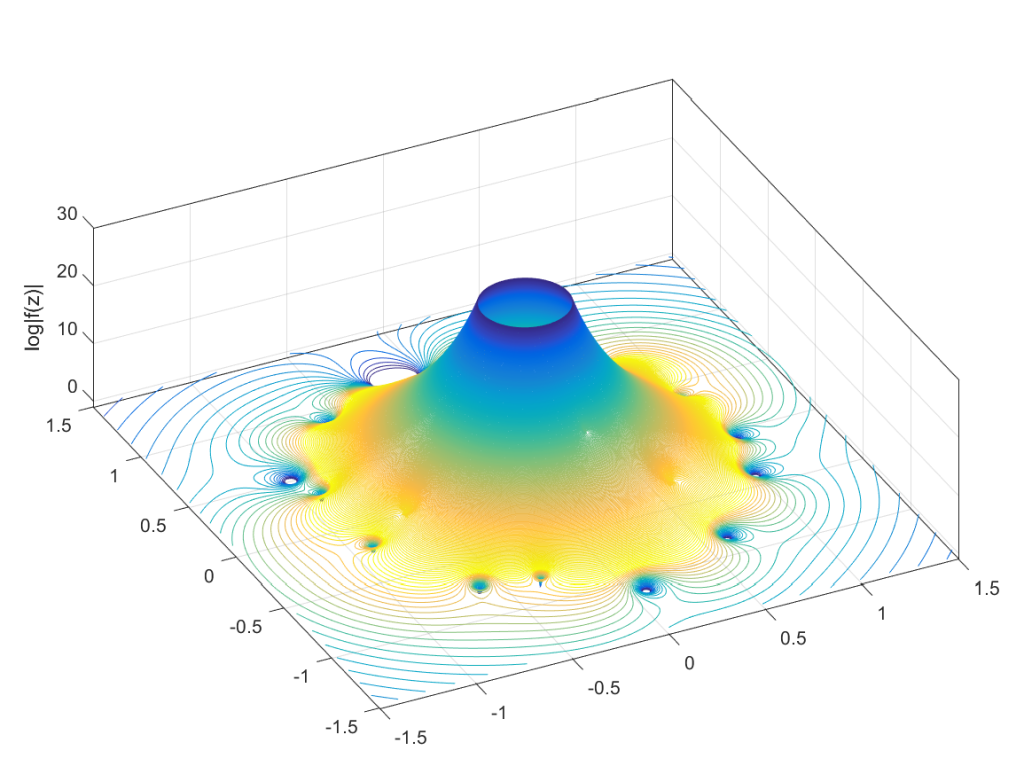Graph of the log-magnitude of f(z), the function mapping a point in the plane to the value of $c_n$ that causes a zero to appear there for $P_n(z)$.

There you have it, a rough theory of dancing zeros.

## References

Harris, G., & Martin, C. (1987). Shorter notes: The roots of a polynomial vary continuously as a function of the coefficients. Proceedings of the American Mathematical Society, 390-392.
Hughes, C. P., & Nikeghbali, A. (2008). The zeros of random polynomials cluster uniformly near the unit circle. Compositio Mathematica, 144(03), 734-746.

# Desperately Seeking Eternity

Overall, I am pretty happy with it (hard to get everything I want into a short essay and without using my academic caveats, footnotes and digressions). Except maybe for the title, since “desperate” literally means “without hope”. You do not seek eternity if you do not hope for anything.

# Adding cooks to the broth

If there are$k$ key ideas needed to produce some important goal (like AI), there is a constant probability per researcher-year to come up with an idea, and the researcher works for$y$ years, what is the the probability of success? And how does it change if we add more researchers to the team?

The most obvious approach is to think of this as y Bernouilli trials with probability p of success, quickly concluding that the number of successes n at the end of y years will be distributed as$\mathrm{Pr}(n)=\binom{y}{n}p^n(1-p)^{y-n}$. Unfortunately, then the actual answer to the question will be$\mathrm{Pr}(n\geq k) = \sum_{n=k}^y \binom{y}{n}p^n(1-p)^{y-n}$ which is a real mess…

A somewhat cleaner way of thinking of the problem is to go into continuous time, treating it as a homogeneous Poisson process. There is a rate$\lambda$ of good ideas arriving to a researcher, but they can happen at any time. The time between two ideas will be exponentially distributed with parameter$\lambda$. So the time$t$ until a researcher has$k$ ideas will be the sum of$k$ exponentials, which is a random variable distributed as the Erlang distribution:$f(t; k,\lambda)=\lambda^k t^{k-1} e^{-\lambda t} / (k-1)!$.

Just like for the discrete case one can make a crude argument that we are likely to succeed if$y$ is bigger than the mean$k/\lambda$ (or$k/p$) we will have a good chance of reaching the goal. Unfortunately the variance scales as$k/\lambda^2$ – if the problems are hard, there is a significant risk of being unlucky for a long time. We have to consider the entire distribution.

Unfortunately the cumulative density function in this case is$\mathrm{Pr}(t which is again not very nice for algebraic manipulation. Still, we can plot it easily.

Before we do that, let us add extra researchers. If there are$N$ researchers, equally good, contributing to the idea generation, what is the new rate of ideas per year? Since we have assumed independence and a Poisson process, it just multiplies the rate by a factor of$N$. So we replace$\lambda$ with$\lambda N$ everywhere and get the desired answer.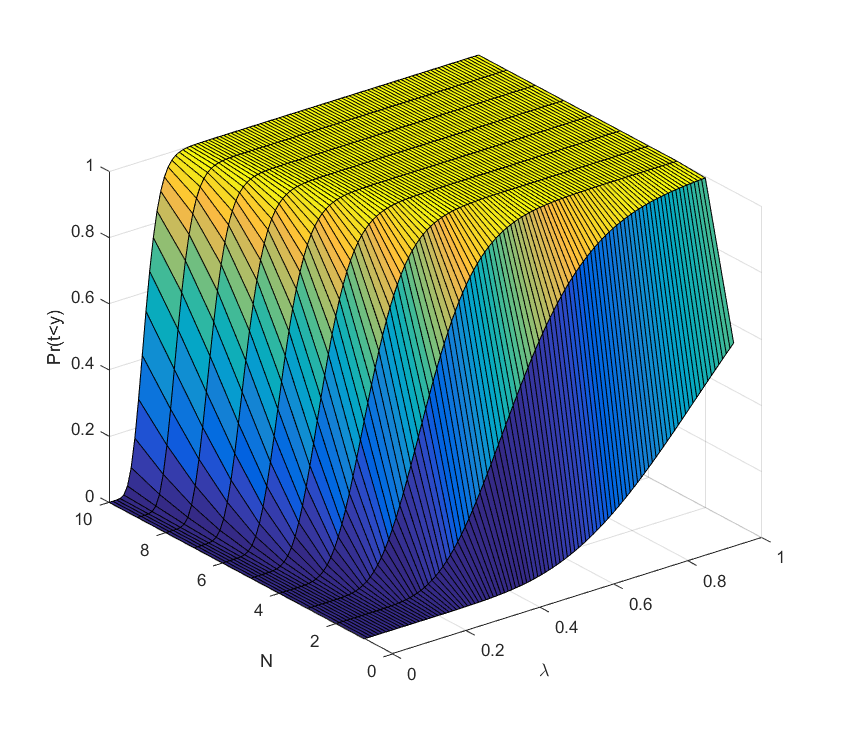This is a plot of the case$k=10, y=10$.

What we see is that for each number of scientists it is a sigmoid curve: if the discovery probability is too low, there is hardly any chance of success, when it becomes comparable to$k/N$ it rises, and sufficiently above we can be almost certain the project will succeed (the yellow plateau). Conversely, adding extra researchers has decreasing marginal returns when approaching the plateau: they make an already almost certain project even more certain. But they do have increasing marginal returns close to the dark blue “floor”: here the chances of success are small, but extra minds increase them a lot.

We can for example plot the ratio of success probability for$\lambda=0.09$ to the one researcher case as we add researchers: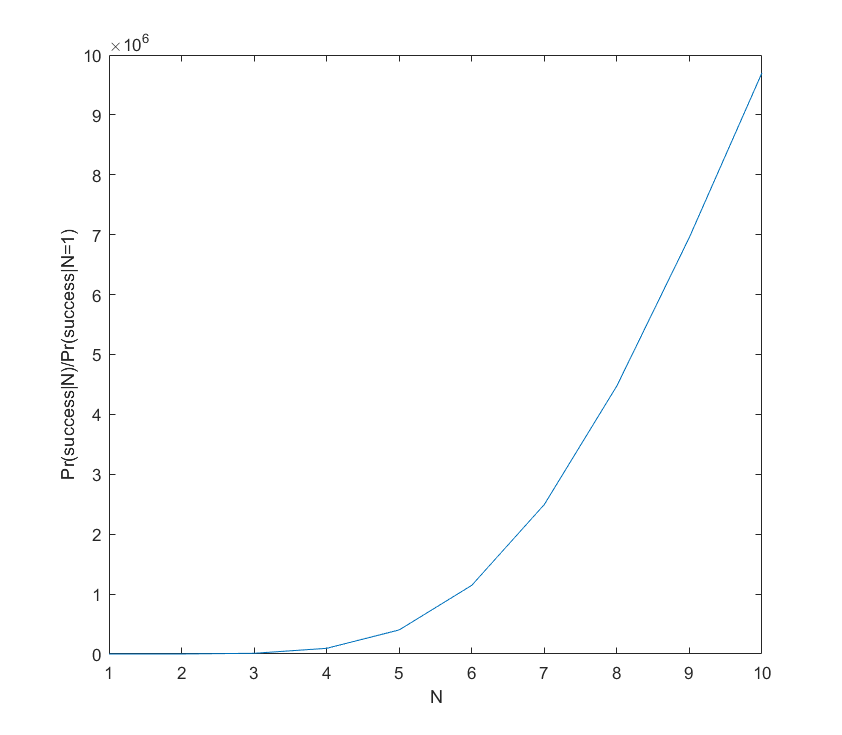Even with 10 researchers the success probability is just 40%, but clearly the benefit of adding extra researchers is positive. The curve is not quite exponential; it slackens off and will eventually become a big sigmoid. But the overall lesson seems to hold: if the project is a longshot, adding extra brains makes it roughly exponentially more likely to succeed.

It is also worth recognizing that in this model time is on par with discovery rate and number of researchers: what matters is the product$\lambda y N$ and how it compares to$k$.

This all assumes that ideas arrive independently, and that there are no overheads for having a large team. In reality these things are far more complex. For example, sometimes you need to have idea 1 or 2 before idea 3 becomes possible: that makes the time$t_3$ of that idea distributed as an exponential plus the distribution of$\mathrm{min}(t_1,t_2)$. If the first two ideas are independent and exponential with rates$\lambda, \mu$, then the minimum is distributed as an exponential with rate$\lambda+\mu$. If they instead require each other, we get a non-exponential distribution (the pdf is$\lambda e^{-\lambda t} + \mu e^{-\mu t} - (\lambda+\mu)e^{-(\lambda+\mu)t}$). Some discoveries or bureaucratic scalings may change the rates. One can construct complex trees of intellectual pathways, unfortunately quickly making the distributions impossible to write out (but still easy to run Monte Carlo on). However, as long as the probabilities and the induced correlations small, I think we can linearise and keep the overall guess that extra minds are exponentially better.

In short: if the cooks are unlikely to succeed at making the broth, adding more is a good idea. If they already have a good chance, consider managing them better.